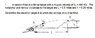# Find the Trajectory's Launch Angle

deveny7

## Homework Statement

A cannon fires at a hilltop target with a muzzle velocity of V = 600 ft/s. The
horizontal and vertical distances to the target are L = 0.5 miles and h = 0.25 miles.
Determine the elevation angle at which the cannon should be fired.

L=2640 ft
h=1320 ft

## Homework Equations

2θ=arcsin([g*R]/V^2)

## The Attempt at a Solution

2θ=asin([32.2*2640]/360000)

2θ=asin(.2361)

θ=6.8282

This would be the correct angle if the cannon ball were to hit the ground, but the ball is landing on the hilltop. What equation should be used to include the quarter mile in the air?

#### Attachments

•HW.jpg
18.4 KB · Views: 396